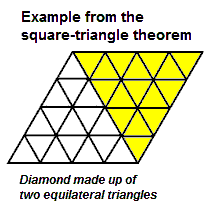# Log24

## Sunday, March 18, 2012

### Square-Triangle Diamond

Filed under: General,Geometry — Tags: — m759 @ 5:01 AM

The diamond shape of yesterday's noon post
is not wholly without mathematical interest …

The square-triangle theorem

"Every triangle is an n -replica" is true
if and only if n  is a square.The 16 subdiamonds of the above figure clearly
may be mapped by an affine transformation
to 16 subsquares of a square array.

(See the diamond lattice  in Weyl's Symmetry .)

Similarly for any square n , not just 16.

There is a group of 322,560 natural transformations
that permute the centers  of the 16 subsquares
in a 16-part square array. The same group may be
viewed as permuting the centers  of the 16 subtriangles
in a 16-part triangular array.